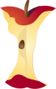Partition Circles and Rectangles In Halves, Quarters, and Other FractionsPrintable Worksheets And Lessons

• Step-by-step Lesson- We teach you the difference between halves and quarters by using a square.

• Guided Lesson - We first ask you how parts shapes are broken into. We move on to ask you how many of those shapes are shaded.

• Guided Lesson Explanation - I find that numbering the parts helps a lot. You might want to get students into that habit.

• Practice Worksheet - Determine the fraction of each shape that is shaded.

• Matching Worksheet - Match the shaded shapes to their numeric or word based fraction.(Click Here to Upgrade)

Homework Sheets

We start by breaking all kinds of common shapes into pieces and parts.

Practice Worksheets

Now we start to ask students to identify the fraction that is right in front of them.

Math Skill Quizzes

These are in the same exact form that have been present on countless State assessments.

 Quiz 1 Quiz 2 Quiz 3